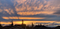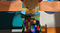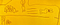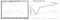# Enable code highlight and math formulas for markdown document

## Code highlight and LaTex math formula in markdown file editing in VSC and post processed by Nodejs.Image by Andrew Zhu, afternoon sunset in Issaquah, WA

## Enable code highlight and Math formulas in Visual Studio Code

````pythondef func():    print("hello python")````

# Ten ways to access Pandas DataFrame, which one is the best?

`import pandas…`

# Measure Text Weight using TF-IDF in Python and scikit-learn

## How we can use TF-IDF to give weights # to text data, and figure out why the result from scikit-learn is different compare with formula from TextbooksImage by Andrew Zhu, my son, Charles’s lego board

# A solution to boost Python speed 1000x timesThe Rabbit And The Turtle — By Charles Zhu, my 6 years old son

## People said Python is slow, how slow it can be

`import numpy as npnp.random.seed(0)values = np.random.randint(1, 100, size=1000000)def get_reciprocal(values):    output = np.empty(len(values))    for i in range(len(values)):        output[i] = 1.0/values[i]%timeit get_reciprocal(values)`
`3.37…`

# Build a User Friendly Chart with MatplotlibBuild a User Friendly Chart with Matplotlib

# Two plotting styles, but which one to use?

`import matplotlib.pyplot as plt(fig, ax) = plt.subplots()ax.plot([1, 2, 3, 4], [1, 4, 2, 3])`
`import matplotlib.pyplot as pltplt.plot([1, …`

# Understand Python import, module, and package

## What happens when PIP install a package

`pip install <pkg_name>`
`/home/<user_name>/.local/lib/python3.x/site-packages`

# The smile of Mona Lisa## Andrew Zhu

Daddy of two kids, husband and Data Scientist @ Microsoft, Redmond.